# US8640014B2 - Soft bit metric generation - Google Patents

## Info

Publication number
US8640014B2
US8640014B2 US13/413,905 US201213413905A US8640014B2 US 8640014 B2 US8640014 B2 US 8640014B2 US 201213413905 A US201213413905 A US 201213413905A US 8640014 B2 US8640014 B2 US 8640014B2
Authority
US
United States
Prior art keywords
including
terms
reliability
dominant terms
dominant
Prior art date
Legal status (The legal status is an assumption and is not a legal conclusion. Google has not performed a legal analysis and makes no representation as to the accuracy of the status listed.)
Active, expires
Application number
US13/413,905
Other versions
US20120230379A1 (en
Inventor
Fan Mo
Sameep Dave
Christian Rasmussen
Mehmet Aydinlik
Current Assignee (The listed assignees may be inaccurate. Google has not performed a legal analysis and makes no representation or warranty as to the accuracy of the list.)
Acacia Communications Inc
Original Assignee
Acacia Communications Inc
Priority date (The priority date is an assumption and is not a legal conclusion. Google has not performed a legal analysis and makes no representation as to the accuracy of the date listed.)
Filing date
Publication date
Priority to US201161449822P priority Critical
Application filed by Acacia Communications Inc filed Critical Acacia Communications Inc
Priority to US13/413,905 priority patent/US8640014B2/en
Assigned to ACACIA COMMUNICATION INCORPORATED reassignment ACACIA COMMUNICATION INCORPORATED ASSIGNMENT OF ASSIGNORS INTEREST (SEE DOCUMENT FOR DETAILS). Assignors: AYDINLIK, MEHMET, RASMUSSEN, CHRISTIAN
Publication of US20120230379A1 publication Critical patent/US20120230379A1/en
Application granted granted Critical
Publication of US8640014B2 publication Critical patent/US8640014B2/en
Active legal-status Critical Current

• 230000000051 modifying Effects 0.000 claims description 11
• 238000004590 computer program Methods 0.000 claims description 9
• 238000000034 methods Methods 0.000 abstract description 7
• 238000004364 calculation methods Methods 0.000 abstract description 3
• 230000000694 effects Effects 0.000 description 1

## Images

•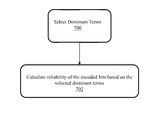•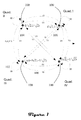•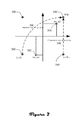•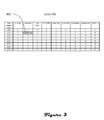•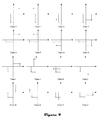•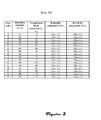••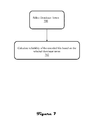## Classifications

• HELECTRICITY
• H04ELECTRIC COMMUNICATION TECHNIQUE
• H04LTRANSMISSION OF DIGITAL INFORMATION, e.g. TELEGRAPHIC COMMUNICATION
• H04L25/00Baseband systems
• H04L25/02Details ; Arrangements for supplying electrical power along data transmission lines
• H04L25/06Dc level restoring means; Bias distortion correction decision circuits providing symbol by symbol detection
• H04L25/067Dc level restoring means; Bias distortion correction decision circuits providing symbol by symbol detection providing soft decisions, i.e. decisions together with an estimate of reliability

## Abstract

Soft bit metric generation computational complexity can be reduced by identifying and utilizing only the dominant terms in a reliability calculation such as a logarithmic likelihood ratio (LLR). The dominant terms are those terms for which the signs of the x and y components match those of channel outputs of the channel outputs. One technique for identifying the dominant terms is by determining the most likely transitions from two consecutive channel output samples Values for the dominant terms can be estimated by either the joint reliability of two consecutive samples of the in-phase component (x1,x2) or by the joint reliability of two consecutive samples of the quadrature components (y1,y2).

## Description

CROSS-REFERENCE TO RELATED APPLICATIONS

Priority is claimed to U.S. Provisional Patent Application Ser. No. 61/449,822, filed Mar. 7, 2011, entitled SOFT BIT METRIC GENERATION FOR DIFFERENTIALLY ENCODED QPSK, which is incorporated by reference.

BACKGROUND OF THE INVENTION

Differential encoding techniques are used by some communications systems to mitigate the undesirable effects of phase noise and channel impairments. Examples include but are not limited to Quadrature Phase Shift Keying (QPSK), Differentially Encoded QPSK (DE-QPSK), 16 Quadrature Amplitude Modulation (QAM) and 64 QAM QPSK is a form of phase shift keying in which two bits are contemporaneously modulated by selecting one of four possible carrier phase shifts (0, π/2, π, and 3π/2). QAM is a modulation technique for conveying either two analog message signals or two digital bit streams by changing the amplitudes of two carrier waves using Amplitude Shift Keying (ASK) or Amplitude Modulation (AM). When these techniques are used, a differential decoding operation is implemented at the receiver to recover the transmitted information. In particular, a “soft decision” decoder uses soft bit metric information to decode data that has been encoded with an error correcting code. The soft bit metric information indicates the reliability of each input data point, and is used to generate a better estimate of the original data than a hard decision decoder would when the data is corrupted by noise. However, soft bit metric information may be computational costly to generate.

SUMMARY OF THE INVENTION

In accordance with one aspect a method comprises: from an expression of reliability of encoded bits associated with a higher order modulation scheme, the expression including a plurality of terms, selecting dominant terms from the plurality of terms; and calculating reliability of the encoded bits based on the selected dominant terms.

In accordance with another aspect an apparatus comprises: circuitry which selects dominant terms from an expression of reliability of encoded bits associated with a higher order modulation scheme; and circuitry which calculates reliability of the encoded bits based on the selected dominant terms.

In accordance with another aspect a computer program stored on a non-transitory computer-readable medium comprises: logic for selecting dominant terms from an expression of reliability of encoded bits associated with a higher order modulation scheme; and logic for calculating reliability of the encoded bits based on the selected dominant terms.

These and other features will be apparent from the detailed description and figures.

BRIEF DESCRIPTION OF THE FIGURES

FIG. 1 illustrates differential encoding.

FIG. 2 illustrates an example transition.

FIG. 3 illustrates a table of possible approximations for correction term c as a function of ∥a|−|b∥.

FIG. 4 illustrates an analysis of the most likely and second most likely transitions over cases A through P, assuming (x1, y1) is always in the third quadrant, and (x2, y2) is moving from 1st quadrant to 4th quadrant in each row.

FIG. 5 illustrates a reliability assignment table.

FIG. 6 illustrates a block diagram of circuitry for soft bit metric generation.

FIG. 7 is a flow diagram of a method for soft bit metric generation.

DETAILED DESCRIPTION

FIG. 1 illustrates differential encoding. Symbols 100 represent DE-QPSK outputs from a differential encoder at rotational positions labeled as (I,Q). Transitions (0°, 90° CW, 90° CCW, 180°) between outputted symbol positions are indicative of input bits denoted as b, b2. For example, from (Ii-1, Qi-1)=(−1/√{square root over (2)},−1/√{square root over (2)}), if the input symbol (b1,b2) to the differential decoder at the next time instant is 00 then no rotation is applied as indicated by transition 102, i.e., to where (Ii,Qi)=(−1/√{square root over (2)},−1/√{square root over (2)}). However, if the input symbol at the next time instant is 01 then 90-degree clockwise rotation is applied as indicated by transition 104, i.e., to where (Ii,Qi)=(−1/√{square root over (2)},1/√{square root over (2)}). If the input symbol at the next time instant is 10 then 90-degree counter-clockwise rotation is applied as indicated by transition 106, i.e., to where (Ii,Qi)=(1/√{square root over (2)},−1/√{square root over (2)}). If the input symbol at the next time instant is 11 then 180-degree counter-clockwise rotation is applied as indicated by transition 108, i.e., to where (Ii,Qi)=(1/√{square root over (2)},1/√{square root over (2)}). The symbols a transmitted on a channel and a receiver observes received values Ct at times t=i−1 and t=i to recover the bits (b1, b2) transmitted at time i.

Soft Decision Encoding/Decoding is used to enhance the accuracy of the receiver, e.g., where the transmitted symbols are distorted by memory-less additive noise and previous and future values of Ct do not provide extra information about the bits. A soft decision decoder operates in response to inputted “soft metrics” which represent the reliability of each encoded bit. If Ct-1 and Ct denote distorted DE-QPSK symbols at the channel output at time instants t−1 and t, respectively, these two symbols can be used to generate the soft metrics for two encoded bits denoted by b1,t and b2,t. The Logarithmic-Likelihood Ratio (LLR) is used for the purposes of this description, but it should be understood that soft metrics can take various other forms which might be utilized. The general equation for the LLR of b1,t is:

$llr ⁡ ( b 1 , t ) = log ⁡ ( P ⁡ ( b i , t = 1 | C t - 1 , ⁢ C t ) P ⁡ ( b i , t = 0 | C t - 1 , ⁢ C t ) ) ( equation ⁢ ⁢ 1 )$
Similarly, the general LLR equation for b2,t can be written as

$llr ⁡ ( b 2 , t ) = log ⁡ ( P ⁡ ( b 2 , t = 1 | C t - 1 , ⁢ C t ) P ⁡ ( b 2 , t = 0 | C t - 1 , ⁢ C t ) ) ( equation ⁢ ⁢ 2 )$
Equation 1 can be expressed as

$llr ⁡ ( b 1 , t ) = log ( P ⁡ ( 00 , 10 | C i - 1 , ⁢ C i ) + P ⁢ ( 00 , 11 | C i - 1 , ⁢ C i ) + P ⁡ ( 01 , 00 | C i - 1 , ⁢ C i ) + P ⁡ ( 01 , 10 | C i - 1 , ⁢ C i ) P ⁡ ( 00 , 00 | C i - 1 , ⁢ C i ) + P ⁡ ( 00 , 01 | C i - 1 , ⁢ C i ) + P ⁡ ( 01 , 01 | C i - 1 , ⁢ C i ) + P ⁡ ( 01 , 11 | C i - 1 , ⁢ C i ) ⁢ ⁢ … ⁢ ⁢ P ⁡ ( 10 , 11 | C i - 1 , ⁢ C i ) + P ⁡ ( 10 , 01 | C i - 1 , ⁢ C i ) + P ⁡ ( 11 , 00 | C i - 1 , ⁢ C i ) + P ⁡ ( 11 , 01 | C i - 1 , ⁢ C i ) P ⁡ ( 10 , 10 | C i - 1 , ⁢ C i ) + P ⁡ ( 10 , 00 | C i - 1 , ⁢ C i ) + P ⁡ ( 11 , 10 | C i - 1 , ⁢ C i ) + P ⁡ ( 11 , 11 | C i - 1 , ⁢ C i ) ) ( equation ⁢ ⁢ 3 )$
P(Ii-1Qi-1,IiQi|Ci-1,Ci) represents the probability of transitioning from Ii-1Qi-1 to IiQi given channel outputs Ct-1 and Ct. Transitions on the numerator of equation 3 are associated with b1,t=1. Transitions on the denominator of equation 3 are associated with b1,t=0. llr(b2,t) can be expressed in a similar way. Assuming that each transmitted DE-QPSK signal has unity energy, the channel only adds Additive White Gaussian Noise, where the noise is complex with 0 mean and variance σ2, Ct-1=(x1,y1), where x1 and y1 denote the in-phase and quadrature components of channel output, respectively, and similarly Ct=(x2,y2), equation 3 can be expressed as:

$llr ⁡ ( b 1 , t ) = log ⁡ ( ⅇ - x 1 - y 1 + x 2 - y 2 σ 2 / 2 + ⅇ - x 1 - y 1 + x 2 + y 2 σ 2 / 2 + ⅇ - x 1 + y 1 - x 2 - y 2 σ 2 / 2 ⁢ + ⅇ - x 1 + y 1 + x 2 - y 2 σ 2 / 2 ⁢ + ⅇ x 1 - y 1 + x 2 + y 2 σ 2 / 2 + ⅇ x 1 - y 1 - x 2 + y 2 σ 2 / 2 + ⅇ x 1 + y 1 - x 2 - y 2 σ 2 / 2 + ⅇ x 1 + y 1 - x 2 + y 2 σ 2 / 2 ⅇ - x 1 - y 1 - x 2 - y 2 σ 2 / 2 + ⅇ - x 1 - y 1 - x 2 + y 2 σ 2 / 2 + ⅇ - x 1 + y 1 - x 2 + y 2 σ 2 / 2 + ⅇ - x 1 + y 1 + x 2 + y 2 σ 2 / 2 + ⅇ x 1 - y 1 + x 2 - y 2 σ 2 / 2 + ⅇ x 1 - y 1 - x 2 - y 2 σ 2 / 2 + ⅇ x 1 + y 1 + x 2 - y 2 σ 2 / 2 + ⅇ x 1 + y 1 + x 2 + y 2 σ 2 / 2 ) ( equation ⁢ ⁢ 4 )$
Also, llr(b2,t) can be written as

$llr ⁡ ( b 2 , t ) = log ⁡ ( ⅇ x 1 + y 1 - x 2 - y 2 σ 2 / 2 + ⅇ x 1 + y 1 + x 2 - y 2 σ 2 / 2 + ⅇ - x 1 + y 1 + x 2 - y 2 σ 2 / 2 + ⅇ - x 1 + y 1 + x 2 + y 2 σ 2 / 2 + ⅇ - x 1 - y 1 + x 2 + y 2 σ 2 / 2 + ⅇ - x 1 - y 1 - x 2 + y 2 σ 2 / 2 + ⅇ x 1 - y 1 - x 2 + y 2 σ 2 / 2 + ⅇ x 1 - y 1 - x 2 - y 2 σ 2 / 2 ⅇ x 1 + y 1 + x 2 + y 2 σ 2 / 2 ⁢ + ⅇ x 1 + y 1 - x 2 + y 2 σ 2 / 2 + ⅇ - x 1 + y 1 - x 2 + y 2 σ 2 ⁢ 2 + ⅇ - x 1 + y 1 - x 2 - y 2 σ 2 / 2 + ⅇ - x 1 - y 1 - x 2 - y 2 σ 2 / 2 + ⅇ - x 1 - y 1 + x 2 - y 2 σ 2 / 2 + ⅇ x 1 - y 1 + x 2 - y 2 σ 2 / 2 + ⅇ x 1 - y 1 + x 2 + y 2 σ 2 / 2 ) ( equation ⁢ ⁢ 5 )$
Although values of equations 4 and 5 can be calculated, each equation includes sixteen terms, each one of which requires the evaluation of an exponential. Therefore, the computational complexity for these equations is relatively high.

In general, computational complexity can be reduced by identifying and utilizing only the dominant terms in a reliability calculation such as the logarithmic likelihood ratio (LLR). The dominant terms in equations 4 and 5 can be selected as, for example, those terms for which the signs of the x and y components match those of channel outputs of the channel outputs. On technique for identifying the dominant terms is by determining the most likely transitions from two consecutive channel output samples that are denoted by Ct-1=(x1,y1) and Ct=(x2,y2), where x and y denote in-phase and quadrature components of the symbol, respectively. [Moved to below] Techniques for reducing computational complexity for soft bit metric generation for differentially encoded higher order modulation schemes will now be described with regard to the example illustrated in FIG. 2.

In the example illustrated in FIG. 2 dots 300 represent the transmitted DE-QPSK symbols denoted by (Ii-1, Qi-1). Dot 302 represents a corresponding noisy channel output received at time instant i−1. Similarly dot 304 denotes a corresponding noisy channel output at time instant i. The most likely transition 306 is (b1, b2)=11, i.e., transition from (Ii-1, Qi-1)=00 to (Ii, Qi)=11. Because x1 has the smallest magnitude among all, the second most likely transition 308 is (b1, b2)=10, i.e., from (Ii-1, Qi-1)=10 to (Ii, Qi)=11, with Ii-1 flipped sign.

In at least one embodiment a “G operation” is used to calculate the reliability of encoded bits (b1, b2) from noisy channel outputs Ct-1=(x1,y1) and Ct=(x2, y2). The G operation computes the joint reliability of two reliability values, which can be either in-phase components (x1, x2) or quadrature components (y1, y2). Given a and b as two reliability values, the G operation can be expressed as:
G(a,b)=min(|a|,|b|)−c(a,b),  (equation 6)
where c(a, b) is a correction term. In the illustrated example the G operation between (x1, x2) is assigned to b2 as the reliability information because the flip of b2 is the most likely event. Conversely, the G operation between (y1, y2) is assigned to b1 as the reliability information.

Hard decision differential decoding can be used to indicate the most likely transition between each pair of received symbols, Ct-1=(x1, y1) and Ct=(x2, y2). In the illustrated example the hard decision differential decoder will return (1,1) since the most likely transition corresponds to (b1, b2)=(1,1).

The signs of the bit reliabilities that are generated using the G operation are determined by hard decision differential decoding. If hard decision decoder returns 1 (0), then positive (negative) signs are assigned to the reliabilities. If the G operation returns 3 for b1 and 4 for b2 then positive signs are assigned to these reliabilities, i.e., llr(b1)=3 and llr(b2)=4.

Referring to FIG. 3, in accordance with one aspect the correction term for the G operation is obtained from a look-up table based on scaling and the signal to noise ratio estimate, e.g., Table 400 (FIG. 4), where c(a,b) is expressed as a function of ∥a|−|b∥ as a further simplification. For example if the SNR estimate is 5.3 dB and ∥a|−|b∥=1, then c(1)=2 is outputted by table 400 as indicated by entry 402.

FIG. 4 illustrates an analysis of the most likely and second most likely transitions over cases A through P, assuming (x1, y1) is always in the third quadrant, and (x2, y2) is moving from 1st quadrant to 4th quadrant in each row. In row 1, x1 has the smallest magnitude for cases A to D. In row 2, y1 has the smallest magnitude for cases E to H. In row three, y2 has the smallest magnitude in the first quadrant for case I, and cases J to L show rotation by 90, 180 and 270 degrees. In row 4, x2 has the smallest magnitude in the first quadrant for case I, and cases N to P show rotation by 90, 180 and 270 degrees. Cases A through P combined cover all relative magnitude possibilities with (x1, y1) in the 3rd quadrant. Referring to FIG. 5, by analyzing cases A through P it can be determined that four of the cases, namely A, C, E and G, shall have the G operation between (x1, x2) assigned to b2 and the G operation between (y1, y2) assigned to b1 as indicated by Table 500. In all other cases the G operation between (x1, x2) should be assigned to b1 and the G operation between (y1, y2) should be assigned to b2. Although only the cases where (x1, y1) is in the third quadrant are described above, all other cases with (x1, y1) in other quadrants will also be analyzed.

FIG. 6 is a block diagram of circuitry for soft bit metric generation. A Hard Decision differential decoder 600 indicates the most likely transition between each pair of received symbols, Ct-1=(x1, y1) and Ct=(x2, y2). The G operation is performed separately on x (x1, x2) and y (y1, y2) components for all cases in table 500 (FIG. 5) using G operation blocks 602, 604. G operation assignment to b1 or b2 is determined by the case index. Consequently, x and y components are applied to G operation blocks 602, 604 separately. A “Case Logic” block 606 determines if the output of two G operations should be swapped by logic 608, 610 based on the case index as described in table 500 (FIG. 5). The Case Logic block 606 computes output g from (x1, x2) and (y1, y2) as:
g=(d 1 ⊕e 1)⊕(f· ((d 1 ⊕e 1)⊕(d 2 ⊕e 2)) ((d 1 ⊕e 1)⊕(d 2 ⊕e 2))  (equation 7)
where

$d n = { 1 , x n ≥ 0 0 , otherwise ( equation ⁢ ⁢ 8 ) e n = { 1 , y n ≥ 0 0 , otherwise ( equation ⁢ ⁢ 9 ) f = { 1 , min ⁡ ( x 1 , y 1 ) < min ⁡ ( x 2 , y 2 ) 0 , otherwise ( equation ⁢ ⁢ 10 )$
and where ⊕; denotes logical XOR and AND operations, respectively. A line drawn above the expression denotes logical NOT. The hard decision differential decoder 600 implements the following equations for the differential encoding scheme:
c 1=(d 1 ⊕d 2)⊕((d 1 ⊕e 1)·( d 2 e 2))
c 2=(e 1 ⊕e 2)⊕((d 1 ⊕e 1)·( e 2 e 2))
where llr(b1) and llr(b2) values are signed by C1 and c2 (Cn=1 indicates a positive number).

FIG. 7 is a flow diagram of a method for soft bit metric generation. The method includes selecting the dominant terms in a reliability calculation such as the logarithmic likelihood ratio (LLR) as indicated by step 700. One technique for identifying the dominant terms is by determining the most likely transitions from two consecutive channel output samples that are denoted by Ct-1(x1, y1) and Ct=(x2, y2), where x and y denote in-phase and quadrature components of the symbol, respectively. The dominant terms in equations 4 and 5 can be selected as, for example, those terms for which the signs of the x and y components match those of channel outputs of the channel outputs. Reliability of the encoded bits is then calculated based on the selected terms as indicated by step 702. Values for the above-mentioned dominant terms can be estimated by either the joint reliability of two consecutive samples of the in-phase component (x1,x2) or by the joint reliability of two consecutive samples of the quadrature components (y1,y2).

It will be appreciated that the invention is not limited to QPSK or other features which have been used to provide context for the describe above. Further, aspects may be implemented using general purpose electronic hardware, purpose-designed electronic hardware, computer program code stored on a non-transitory computer-readable medium and operated upon by a general or purpose-designed processor, or any combinations thereof. Furthermore, while the invention is described through the above exemplary embodiments, it will be understood by those of ordinary skill in the art that modification to and variation of the illustrated embodiments may be made without departing from the inventive concepts herein disclosed. Moreover, while the preferred embodiments are described in connection with various illustrative structures, one skilled in the art will recognize that the system may be embodied using a variety of specific structures. Accordingly, the invention should not be viewed as limited except by the scope and spirit of the appended claims.

## Claims (21)

What is claimed is:
1. A method comprising:
from an expression of reliability of encoded bits associated with a higher order modulation scheme, the expression including a plurality of terms, selecting dominant terms from the plurality of terms; and
calculating reliability of the encoded bits based on the selected dominant terms.
2. The method of claim 1 including identifying the dominant terms by determining the most likely transitions from two consecutive channel output samples.
3. The method of claim 1 including approximating the dominant terms based on joint reliability of two consecutive samples of an in-phase component.
4. The method of claim 1 including approximating the dominant terms based on joint reliability of two consecutive samples of quadrature components.
5. The method of claim 1 including approximating the dominant terms based on joint reliability with a function G(a, b)=min(|a|,|b|)−c(a, b).
6. The method of claim 5 including obtaining correction term c(a, b) from a set of pre-calculated terms.
7. The method of claim 1 including determining a sign to associate with a soft metric from hard decision differential decoding.
8. Apparatus comprising:
circuitry which selects dominant terms from an expression of reliability of encoded bits associated with a higher order modulation scheme; and
circuitry which calculates reliability of the encoded bits based on the selected dominant terms.
9. The apparatus of claim 8 including circuitry which identifies the dominant terms by determining the most likely transitions from two consecutive channel output samples.
10. The apparatus of claim 8 including circuitry which approximates the dominant terms based on joint reliability of two consecutive samples of an in-phase component.
11. The apparatus of claim 8 including circuitry which approximates the dominant terms based on joint reliability of two consecutive samples of quadrature components.
12. The apparatus of claim 8 including circuitry which approximates the dominant terms based on joint reliability with a function G(a, b)=min(|a,|b|)−c(a, b).
13. The apparatus of claim 12 including circuitry which obtains correction term c(a, b) from a set of pre-calculated terms.
14. The apparatus of claim 8 including circuitry which determines a sign to associate with a soft metric from hard decision differential decoding.
15. A computer program stored on a non-transitory computer-readable medium comprising:
logic for selecting dominant terms from an expression of reliability of encoded bits associated with a higher order modulation scheme; and
logic for calculating reliability of the encoded bits based on the selected dominant terms.
16. The computer program of claim 15 including logic for identifying the dominant terms by determining the most likely transitions from two consecutive channel output samples.
17. The computer program of claim 15 including logic for approximating the dominant terms based on joint reliability of two consecutive samples of an in-phase component.
18. The computer program of claim 15 including logic for approximating the dominant terms based on joint reliability of two consecutive samples of quadrature components.
19. The computer program of claim 15 including logic for approximating the dominant terms based on joint reliability with a function G(a, b)=min(|a,|b|)−c(a, b).
20. The computer program of claim 19 including logic for obtaining correction term c(a, b) from a set of pre-calculated terms.
21. The computer program of claim 15 including logic for determining a sign to associate with a soft metric from hard decision differential decoding.
US13/413,905 2011-03-07 2012-03-07 Soft bit metric generation Active 2032-07-25 US8640014B2 (en)

## Priority Applications (2)

Application Number Priority Date Filing Date Title
US201161449822P true 2011-03-07 2011-03-07
US13/413,905 US8640014B2 (en) 2011-03-07 2012-03-07 Soft bit metric generation

## Applications Claiming Priority (1)

Application Number Priority Date Filing Date Title
US13/413,905 US8640014B2 (en) 2011-03-07 2012-03-07 Soft bit metric generation

## Publications (2)

Publication Number Publication Date
US20120230379A1 US20120230379A1 (en) 2012-09-13
US8640014B2 true US8640014B2 (en) 2014-01-28

# Family

## Family Applications (1)

Application Number Title Priority Date Filing Date
US13/413,905 Active 2032-07-25 US8640014B2 (en) 2011-03-07 2012-03-07 Soft bit metric generation

## Country Status (2)

US (1) US8640014B2 (en)
WO (1) WO2012122249A2 (en)

## Families Citing this family (1)

* Cited by examiner, † Cited by third party
Publication number Priority date Publication date Assignee Title
US9843414B2 (en) * 2014-07-01 2017-12-12 Utah State University Low complexity error correction

## Citations (5)

* Cited by examiner, † Cited by third party
Publication number Priority date Publication date Assignee Title
US5805977A (en) * 1996-04-01 1998-09-08 Motorola, Inc. Method and apparatus for controlling transmissions in a two-way selective call communication system
US5930272A (en) * 1997-06-10 1999-07-27 Efficient Channel Coding, Inc. Block decoding with soft output information
US6697441B1 (en) 2000-06-06 2004-02-24 Ericsson Inc. Baseband processors and methods and systems for decoding a received signal having a transmitter or channel induced coupling between bits
US20060126761A1 (en) 2002-11-22 2006-06-15 Bo Bernhardsson Calculation of soft decision values using reliability information of the amplitude
US7623602B2 (en) * 2005-11-15 2009-11-24 Tensorcomm, Inc. Iterative interference canceller for wireless multiple-access systems employing closed loop transmit diversity

## Patent Citations (5)

* Cited by examiner, † Cited by third party
Publication number Priority date Publication date Assignee Title
US5805977A (en) * 1996-04-01 1998-09-08 Motorola, Inc. Method and apparatus for controlling transmissions in a two-way selective call communication system
US5930272A (en) * 1997-06-10 1999-07-27 Efficient Channel Coding, Inc. Block decoding with soft output information
US6697441B1 (en) 2000-06-06 2004-02-24 Ericsson Inc. Baseband processors and methods and systems for decoding a received signal having a transmitter or channel induced coupling between bits
US20060126761A1 (en) 2002-11-22 2006-06-15 Bo Bernhardsson Calculation of soft decision values using reliability information of the amplitude
US7623602B2 (en) * 2005-11-15 2009-11-24 Tensorcomm, Inc. Iterative interference canceller for wireless multiple-access systems employing closed loop transmit diversity

## Also Published As

Publication number Publication date
US20120230379A1 (en) 2012-09-13
WO2012122249A2 (en) 2012-09-13
WO2012122249A3 (en) 2014-04-17

## Similar Documents

Publication Publication Date Title
KR100770189B1 (en) Method and apparatus for computing soft decision input metrics to a turbo decoder
US6904097B2 (en) Method and apparatus for adaptive signaling in a QAM communication system
US7924932B2 (en) Decoding system and method for digital communications
US7289577B2 (en) Modulation method and radio communication system
US7076000B2 (en) Soft-decision metric generation for higher order modulation
US5233630A (en) Method and apparatus for resolving phase ambiguities in trellis coded modulated data
US8160175B2 (en) Quasi-pilot symbol substitution
US6434200B1 (en) TCM revisiting system and method
JP5111759B2 (en) System and method for forward error correction decoding using soft information
KR100944836B1 (en) Data detection for a hierarchical coded data transmission
US6216107B1 (en) High-performance half-rate encoding apparatus and method for a TDM system
JP4575982B2 (en) 16QAM signal space expansion
US7145966B2 (en) Signal quality estimation for continuous phase modulation
EP1946509B1 (en) Method and apparatus for normalizing input metric to a channel decoder in a wireless communication system
US20110014865A1 (en) Cooperative reception diversity apparatus and method based on signal point rearrangement or superposition modulation in relay system
JP2978792B2 (en) Soft decision method and receiver
EP1262027B1 (en) Method of differential coding and modulation
JP3926499B2 (en) Convolutional code soft decision decoding receiver
US9191044B1 (en) Methods and apparatus for multiple input multiple output (MIMO) successive interference cancellation (SIC)
KR100651570B1 (en) Methdo and apparatus for calculating log likelihood ratio for decoding in a receiver of a mobile communication system
US6661282B2 (en) Demodulation apparatus and method in a communication system employing 16-ary QAM
US8873684B2 (en) BICM decoding in the presence of co-channel interference
US7418060B2 (en) Improving hierarchical 8PSK performance
US7529323B2 (en) Quaternary precoded continuous phase modulation soft bit metric demodulator

## Legal Events

Date Code Title Description
AS Assignment

Owner name: ACACIA COMMUNICATION INCORPORATED, MASSACHUSETTS

Free format text: ASSIGNMENT OF ASSIGNORS INTEREST;ASSIGNORS:RASMUSSEN, CHRISTIAN;AYDINLIK, MEHMET;REEL/FRAME:028246/0381

Effective date: 20120508

STCF Information on status: patent grant

Free format text: PATENTED CASE

FPAY Fee payment

Year of fee payment: 4